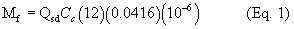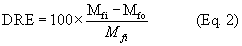# Title 40

## SECTION 63.9323

### 63.9323 How do I determine the add-on control device emission destruction or removal efficiency

§ 63.9323 How do I determine the add-on control device emission destruction or removal efficiency?

You must use the procedures and test methods in this section to determine the add-on control device emission destruction or removal efficiency as part of the performance test required by § 63.9310. You must conduct three test runs as specified in § 63.7(e)(3), and each test run must last at least 1 hour.

(a) For all types of add-on control devices, use the test methods specified in paragraphs (a)(1) through (5) of this section.

(1) Use Method 1 or 1A of appendix A to 40 CFR part 60, as appropriate, to select sampling sites and velocity traverse points.

(2) Use Method 2, 2A, 2C, 2D, 2F, or 2G of appendix A to 40 CFR part 60, as appropriate, to measure gas volumetric flow rate.

(3) Use Method 3, 3A, or 3B of appendix A to 40 CFR part 60, as appropriate, for gas analysis to determine dry molecular weight. The ANSI/ASME PTC 19.10-1981 Part 10 is an acceptable alternative to Method 3B (incorporated by reference, see § 63.14).

(4) Use Method 4 of appendix A to 40 CFR part 60, to determine stack gas moisture.

(5) Methods for determining gas volumetric flow rate, dry molecular weight, and stack gas moisture must be performed, as applicable, during each test run.

(b) Measure total gaseous organic mass emissions as carbon at the inlet and outlet of the add-on control device simultaneously, using either Method 25 or 25A of appendix A to 40 CFR part 60, as specified in paragraphs (b)(1) through (3) of this section. You must use the same method for both the inlet and outlet measurements.

(1) Use Method 25 of appendix A to 40 CFR part 60 if the add-on control device is an oxidizer, and you expect the total gaseous organic concentration as carbon to be more than 50 parts per million at the control device outlet.

(2) Use Method 25A of appendix A to 40 CFR part 60 if the add-on control device is an oxidizer, and you expect the total gaseous organic concentration as carbon to be 50 ppm or less at the control device outlet.

(c) For each test run, determine the total gaseous organic emissions mass flow rates for the inlet and the outlet of the add-on control device, using Equation 1 of this section. If there is more than one inlet or outlet to the add-on control device, you must calculate the total gaseous organic mass flow rate using Equation 1 of this section for each inlet and each outlet and then total all of the inlet emissions and total all of the outlet emissions.Where: Mf = total gaseous organic emissions mass flow rate, kg/hour (kg/h) Cc = concentration of organic compounds as carbon in the vent gas, as determined by Method 25 or Method 25A, parts per million by volume (ppmv), dry basis Qsd = volumetric flow rate of gases entering or exiting the add-on control device, as determined by Method 2, 2A, 2C, 2D, 2F, or 2G, dry standard cubic meters/hour (dscm/h) 0.0416 = conversion factor for molar volume, kg-moles per cubic meter (mol/m 3) (@ 293 Kelvin [K] and 760 millimeters of mercury [mmHg]).

(d) For each test run, determine the add-on control device organic emissions destruction or removal efficiency, using Equation 2 of this section:Where: DRE = organic emissions destruction or removal efficiency of the add-on control device, percent Mfi = total gaseous organic emissions mass flow rate at the inlet(s) to the add-on control device, using Equation 1 of this section, kg/h Mfo = total gaseous organic emissions mass flow rate at the outlet(s) of the add-on control device, using Equation 1 of this section, kg/h.

(e) Determine the emission destruction or removal efficiency of the add-on control device as the average of the efficiencies determined in the three test runs and calculated in Equation 2 of this section.